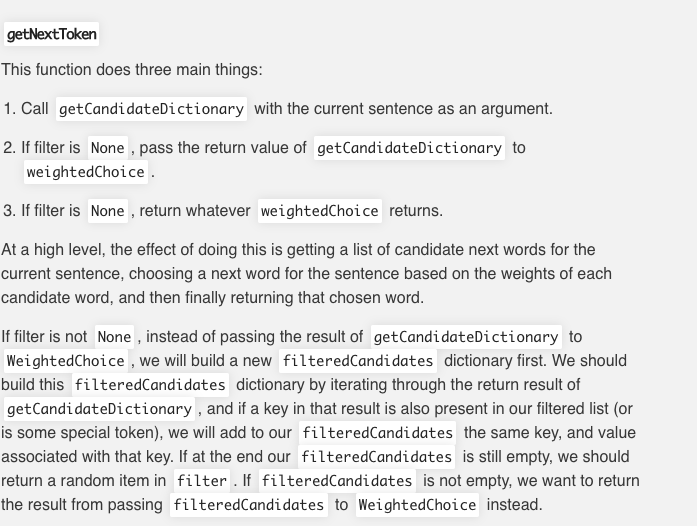# (Solved) : Write Code Fordef Getnexttoken Self Sentence Filter None Import Randomfrom Creativeaidatad Q42720823 . . .

How to write the code for def getNextToken(self, sentence, filter=None)?import randomfrom creative_ai.data.dataLoader import prepDatafrom creative_ai.models.unigramModel import UnigramModelfrom creative_ai.models.bigramModel import BigramModelfrom creative_ai.models.trigramModel import TrigramModelfrom creative_ai.utils.print_helpers import key_value_pairsclass LanguageModel(): def __init__(self, models=None): “”” Requires: nothing Modifies: self (this instance of the LanguageModel object) Effects: This is the LanguageModel constructor. It sets up an empty dictionary as a member variable. This function is done for you. “”” if models != None: self.models = models else: self.models = [TrigramModel(), BigramModel(), UnigramModel()] def __str__(self): “”” Requires: nothing Modifies: nothing Effects: This is a string overloaded. This function is called when languageModel is printed. It will show the number of trained paths for each model it contains. It may be useful for testing. This function is done for you. “”” output_list = [ ‘{} contains {} trained paths.’.format( model.__class__.__name__, key_value_pairs(model.nGramCounts) ) for model in self.models ] output = ‘n’.join(output_list) return output def updateTrainedData(self, text, prepped=True): “”” Requires: text is a 2D list of strings Modifies: self (this instance of the LanguageModel object) Effects: adds new trained data to each of the languageModel models. If this data is not prepped (prepped==False) then it is prepepd first before being passed to the models. This function is done for you. “”” if (not prepped): text = prepData(text) for model in self.models: model.trainModel(text)################################################################################ Begin Core >> FOR CORE IMPLEMENTION, DO NOT EDIT ABOVE OF THIS SECTION <<############################################################################### def selectNGramModel(self, sentence): “”” Requires: self.models is a list of NGramModel objects sorted by descending priority: tri-, then bi-, then unigrams. sentence is a list of strings. Modifies: nothing Effects: returns the best possible model that can be used for the current sentence based on the n-grams that the models know. (Remember that you wrote a function that checks if a model can be used to pick a word for a sentence!) “”” pass def weightedChoice(self, candidates): “”” Requires: candidates is a dictionary; the keys of candidates are items you want to choose from and the values are integers Modifies: nothing Effects: returns a candidate item (a key in the candidates dictionary) based on the algorithm described in the spec. “”” index = [] token = [] count = [] cumulative = [] total = 0 index.append(total) for num in count: total += 1 index.append(total) for tokenNumber in candidates.keys(): token.append(tokenNumber) for countNumber in candidates.values(): count.append(countNumber) sum = 0 for i in count: sum += i cumulative.append(sum) randomNum = random.randrange(0,10) for index, j in enumerate(cumulative): if randomNum < j: return token[index] def getNextToken(self, sentence, filter=None): “”” Requires: sentence is a list of strings, and this model can be used to choose the next token for the current sentence Modifies: nothing Effects: returns the next token to be added to sentence by calling the getCandidateDictionary and weightedChoice functions. For more information on how to put all these functions together, see the spec. If a filter is being used, and none of the models can produce a next token using the filter, then a random token from the filter is returned instead. “””getNextToken This function does three main things: 1. Call getCandidateDictionary with the current sentence as an argument. 2. If filter is None , pass the return value of getCandidateDictionary to weightedChoice 3. If filter is None , return whatever weightedChoice returns. At a high level, the effect of doing this is getting a list of candidate next words for the current sentence, choosing a next word for the sentence based on the weights of each candidate word, and then finally returning that chosen word. If filter is not None , instead of passing the result of getCandidateDictionary to WeightedChoice , we will build a new filtered Candidates dictionary first. We should build this filtered Candidates dictionary by iterating through the return result of getCandidateDictionary, and if a key in that result is also present in our filtered list (or is some special token), we will add to our filteredCandidates the same key, and value associated with that key. If at the end our filtered Candidates is still empty, we should return a random item in filter. If filtered Candidates is not empty, we want to return the result from passing filtered Candidates to WeightedChoice instead. Show transcribed image text getNextToken This function does three main things: 1. Call getCandidateDictionary with the current sentence as an argument. 2. If filter is None , pass the return value of getCandidateDictionary to weightedChoice 3. If filter is None , return whatever weightedChoice returns. At a high level, the effect of doing this is getting a list of candidate next words for the current sentence, choosing a next word for the sentence based on the weights of each candidate word, and then finally returning that chosen word. If filter is not None , instead of passing the result of getCandidateDictionary to WeightedChoice , we will build a new filtered Candidates dictionary first. We should build this filtered Candidates dictionary by iterating through the return result of getCandidateDictionary, and if a key in that result is also present in our filtered list (or is some special token), we will add to our filteredCandidates the same key, and value associated with that key. If at the end our filtered Candidates is still empty, we should return a random item in filter. If filtered Candidates is not empty, we want to return the result from passing filtered Candidates to WeightedChoice instead.

Answer to How to write the code for def getNextToken(self, sentence, filter=None)? import random from creative_ai.data.dataLoader …

We are the best freelance writing portal. Looking for online writing, editing or proofreading jobs? We have plenty of writing assignments to handle.